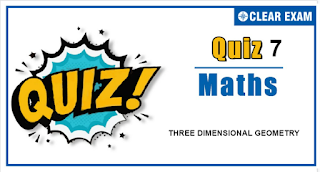## Three Dimensional Geometry Quiz

Important topics for Maths has been designed in such a way that it offers very practical and application-based learning to further make it easier for students to understand every concept or topic by correlating it with the day-to-day experiences.

Q1. The radius of the circle in which the sphere x^2+y^2+z^2+2z-2y-4z-19=0 is cut by the plane x+2y+2z+7=0 is
•  2
•  3
•  4
•  1
Solution
Centre of the sphere is (-1,1,2) and its radius =√(1+1+4+19)=5 CL, perpendicular distance of C from plane, is |(-1+2+4+7)/√(1+4+4)|=4 Now AL^2=CA^2-CL^2=25-16=9 Hence, radius of the circle =√9=3

Q2.The image of the point (-1,3,4) in the plane x-2y=0 is
•  (-17/3,-19/3,4)
•  (15,11, 4)
•  (-17/3,-19/3,1)
•  (9/5,-13/5,4)
Solution
(d) Let P(α,β,γ) be the image of the point Q(-1,3,4) Midpoint of PQ lies on x-2y=0. Then, (α-1)/2-2((β+3)/2)=0 ⇒ α-1-2β-6=0 ⇒ α-2β=7 (i) Also PQ is perpendicular to the plane. Then, (α+1)/1=(β-3)/(-2)=(γ-4)/0 (ii) Solving (i) and (ii), we get α=9/5,β=-13/5,γ=4 Therefore, image is (9/5,-13/5,4) Alternative method: For image, (α-(-1))/1=(β-3)/(-2)=(γ-4)/0=(-2(-1-2(3)))/((1)^2+(-2)^2 ) ⇒ α=9/5,β=-13/5,γ=4

Q3.  If the foot of the perpendicular from the origin to a plane is P(a,b,c), the equation of the plane is
•   x/a+y/b+z/c=3
•  ax+by+cz=3
•  ax+by+cz=a^2+b^2+c^2
•  ax+by+cz=a+b+c
Solution
(c) Direction ratios of OP are (a,b,c) Therefore, equation of the plane is a(x-a)+b(y-b)+c(z-c)=0 i.e., xa+yb+zc=a^2+b^2+c^2

Q4. The equation of the plane passing through the lines (x-4)/1=(y-3)/1=(z-2)/2 and (x-3)/1=(y-2)/(-4)=z/5 is
•  11x-y-3z=35
•  11x+y-3z=35
•  11x-y+3z=35
•  None of these
Solution
(d) Here, the required plane is a(x-4)+b(y-3)+c(z-2)=0 Also a+b+2c=0 and a-4b+5c=0 Solving, we have a/(5+8)=b/(2-5)=c/(-4-1)=k a/13=b/(-3)=c/(-5)=k Therefore, the required equation of plane is -13x+3y+5z+33=0

Q5. Find the equation of a straight line in the plane r ∙n =d which is parallel to r=a +λ b and passes through the foot of the perpendicular drawn from point P(a ) to r ∙n =d (where n ∙b =0)
•  r =a +((d-a ∙n )/n^2 ) n +λ b
•  r =a +((d-a ∙n )/n) n +λ b
•  r =a +((a ∙n -d)/n^2 ) n +λ b ⃗
•  r =a +((a ∙n -d)/n) n +λ b
Solution
Foot of the perpendicular drawn from point A(a) on the plane r .n =d is a+((d-a.n)/〖|n|〗^2 ) n Therefore, equation of the line parallel to r =a +λb ⃗ in the plane r .n =d is given by r =a +((d-a.n)/〖|n|〗^2 ) n +λb

Q6. A plane passes through (1,-2,1) and is perpendicular to two planes 2x-2y+z=0 and x-y+2z=4, then the distance of the plane from the point (1,2,2)is
•  0
•  1
• √2
•  2√2
Solution
(d) Let the equation of plane be, a(x-1)+b(y+2)+c(z-1)=0 Which is perpendicular to 2x-2y+z=0 and x-y+2z=4 ∴2a-2b+c=0 and a-b+2c=0 ⟹a/(-3)=b/(-3)=c/0 ⟹a/1=b/1=c/0 ∴ The equation of plane is, 1(x-1)+1(y+2)+0(z-1)=0 ⟹x+y+1=0,its distance from the point (1,2,2)is (|1+2+1|)/√2=2√2

Q7. A straight line L on the xy-plane bisects the angle between OX and OY. What are the direction cosines of L?
•  <(1/√2),(1/√2),0>
•  <(1/2),(√3/2),0>
•  <0,0,1>
•  <(2/3),(2/3),(1/3)>
Solution
(a) The given line makes angles of π/4,π/4, and π/2 with the x-,y- and z-axes, respectively, ⇒ Direction cosines of the given line are cos⁡(π/4),cos⁡(π/4) and cos⁡(π/2), or (1/√2),(1/√2) and 0

Q8.A unit vector parallel to the intersection of the plane r ∙(i ̂-j ̂+k ̂ )=5 and r ∙(2i ̂+j ̂-3k ̂ )=4 is
•  (2i ̂+5j ̂-3k ̂)/√38
•  (2i ̂-5j ̂+3k ̂)/√38
•  (-2i ̂-5j ̂-3k ̂)/√38
•  (-2i ̂+5j ̂-3k ̂)/√38
Solution
(c) a ̂=±(n _1×n _2)/|n _1×n _2 | =±(2i ̂+5j ̂+3k ̂)/√38 (where n_1 and n_2 are normal to the planes)

Q9. If the plane x/2+y/3+z/6=1 cuts the axes of coordinates at points A,B and C, then find the area of then triangle ABC
•  18 sq unit
•  36 sq unit
•  3√14 sq unit
•  2√14 sq unit
Solution
(c) Plane meets axes at A(2,0,0),B(0,3,0) and C(0,0,6) Then area of ∆ABC is 1/2 |(AB) ×(AC) | =1/2 |(-2i ̂+3j ̂ )×(-2i ̂+6j ̂ )| =3√14 sq units

Q10. The ratio in which the line segment joining the points whose position vectors are 2i ̂-4j ̂-7k ̂ and -3i ̂+5j ̂-8k ̂ is divided by the plane whose equation is r ̂.(i ̂-2j ̂+3k ̂ )=13, is
•  13:12 internally
•  12:25 externally
•  13:25 internally
• 37:25 internally
Solution
(b) Let P be the point and it divides the line segment in the ratio λ:1. Then, (OP) =r =(-3λ+2)/(λ+1) i ̂+(5λ-4)/(λ+1) j ̂+(-8λ-7)/(λ+1) k ̂ It satisfies r .(i ̂-2j ̂+3k ̂ )=13. So, (-3λ+2)/(λ+1)-2 (5λ-4)/(λ+1)+3 (-8λ-7)/(λ+1)=13 or -3λ+2-2(5λ-4)+3(-8λ-7)=13(λ+1) or -37λ-11=13λ+13 or 50λ=-24 or λ=-12/25#### Written by: AUTHORNAME

AUTHORDESCRIPTION## Want to know more

Please fill in the details below:

## Latest NEET Articles\$type=three\$c=3\$author=hide\$comment=hide\$rm=hide\$date=hide\$snippet=hide

Name

ltr
item
BEST NEET COACHING CENTER | BEST IIT JEE COACHING INSTITUTE | BEST NEET & IIT JEE COACHING: Three-Dimensional-Geometry-Quiz-7
Three-Dimensional-Geometry-Quiz-7
https://1.bp.blogspot.com/-Ko-OWychB-s/YNtsEwG4qrI/AAAAAAAAO1A/2YdLi1SepXchPI0Q5dYQaq0qSnvfmwKhACLcBGAsYHQ/s320/QUIZ7.PNG
https://1.bp.blogspot.com/-Ko-OWychB-s/YNtsEwG4qrI/AAAAAAAAO1A/2YdLi1SepXchPI0Q5dYQaq0qSnvfmwKhACLcBGAsYHQ/s72-c/QUIZ7.PNG
BEST NEET COACHING CENTER | BEST IIT JEE COACHING INSTITUTE | BEST NEET & IIT JEE COACHING
https://www.cleariitmedical.com/2021/07/three-dimensional-geometry-quiz-7.html
https://www.cleariitmedical.com/
https://www.cleariitmedical.com/
https://www.cleariitmedical.com/2021/07/three-dimensional-geometry-quiz-7.html
true
7783647550433378923
UTF-8

STAY CONNECTED